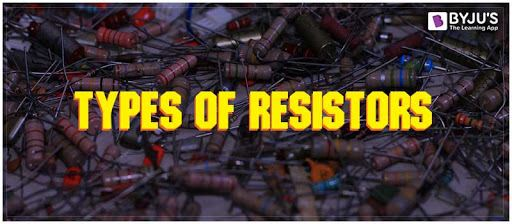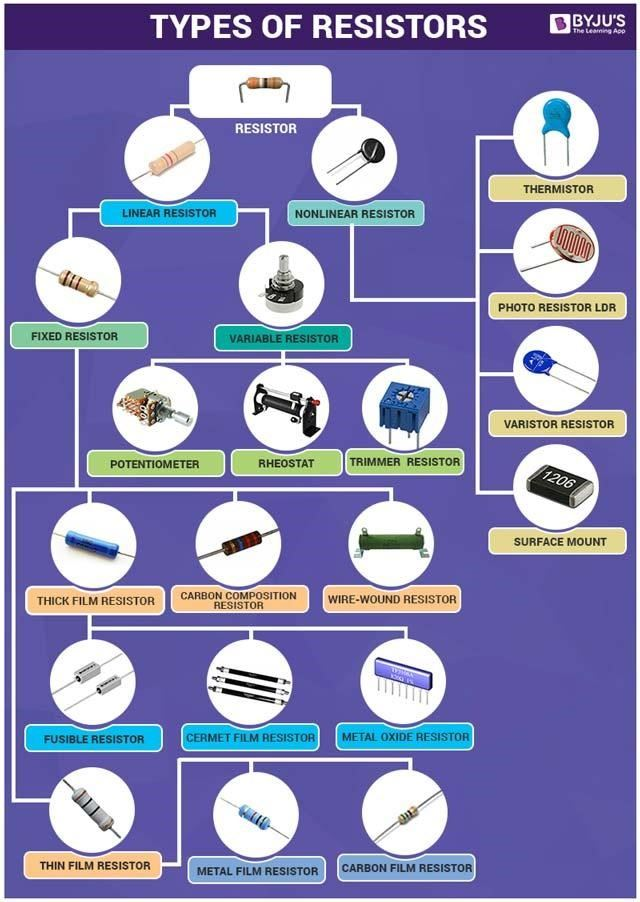# Types Of Resistors## What is a Resistor?

A resistor is a device that obstructs the flow of current. It is a passive two-terminal device which is used to regulate the flow of electric current. Glass, Mica, Wood, Rubber, etc. are examples of resistive materials. The unit of resistance is OHM (Ω) where 1Ω = 1V/1A. In electronic circuits, resistors are used to adjust signal levels, reduce current flow, bias active elements, terminate transmission lines, divide voltages, etc.

## Types Of ResistorsThere are numerous types of resistors that are available and can be used in electronic circuits. These different types of resistors have different properties depending upon their manufacture and construction. There are different types of resistors available for various applications. The resistors are available in different shapes, size, and materials. Normally resistors can be classified into two types namely linear resistor and non-linear resistor.

## Linear Resistors

The resistors whose value changes with the applied temperature and voltage, are called linear resistors. Most types of resistors are linear devices that produce a voltage drop across themselves when a current flows through them There are two basic types of resistors with linear properties namely fixed resistors and variable resistors.

### Fixed resistors

Fixed resistors are resistors with a specific value. Fixed resistors one of the most widely used types of resistor. Fixed resistors are used in electronics circuits to set the correct conditions in a circuit.

### Types of Fixed Resistors

1. Wire Wound Resistors
2. Thin Film Resistors
3. Carbon Composition Resistors

### Variable Resistors

Variable Resistors consist of a slider which taps onto the main resistor element and a fixed resistor element. Simply we can say that a variable resistor is a potentiometer with only 2 connecting wires instead of 3.

### Types of Variable Resistors

1. Potentiometer
2. Rheostat
3. Trimmer Resistor

Following is the table explaining other related concepts of resistors:

## Non-Linear Resistor

Non-linear resistors are those types of resistors where the electric current flowing through it changes with the exchange in applied voltage or temperature and does not change according to Ohm’s law. There are several types of non-linear resistors, but the most commonly used are mentioned below.

### Thermistors

Thermistors are a type of variable resistor that notices the change in temperature. In other words, it is a 2 terminal device that is very sensitive to temperature. The Resistance of a Thermistor is inversely proportional to the temperature.

### Varistor Resistors

A varistor is a non-linear resistor made of semiconductor and current through which depends non-linearly on the applied voltage across it. MOV or metal oxide varistor is the most commonly used form of Varistor Resistors.

### Photo Resistor or LDR (Light Dependent Resistors)

Photo Resistor or LDR (Light Dependent Resistors) or Photo Conductive Cell is a light-controlled variable resistor. The photo resistor resistance decreases with increase in incident light intensity.

### Surface Mount Resistors

Surface Mount Resistors also called SMD resistor are rectangular in shape. An SMD is an electronic component that is made to use with SMT or Surface Mount Technology.